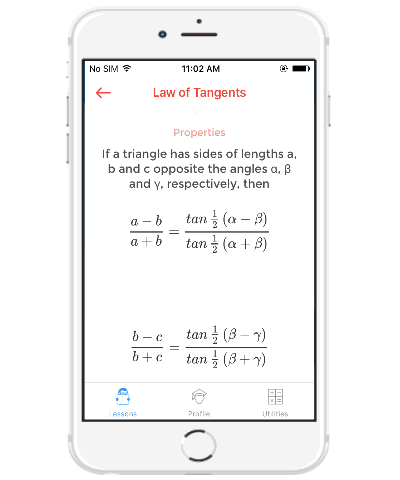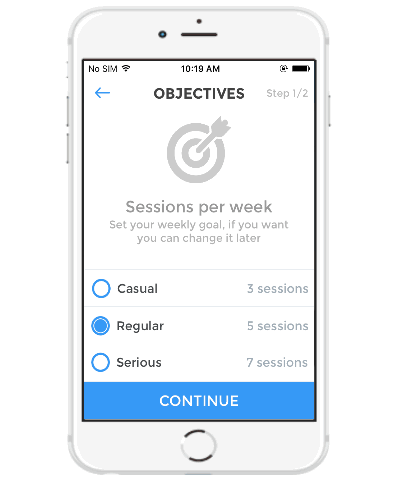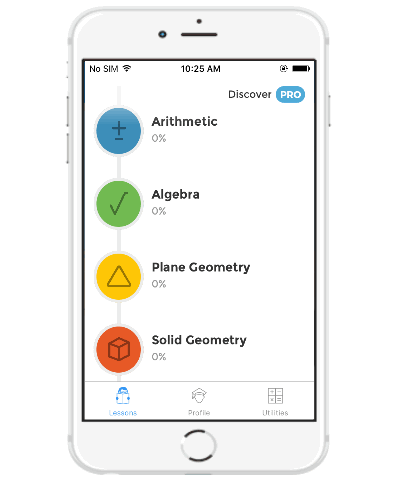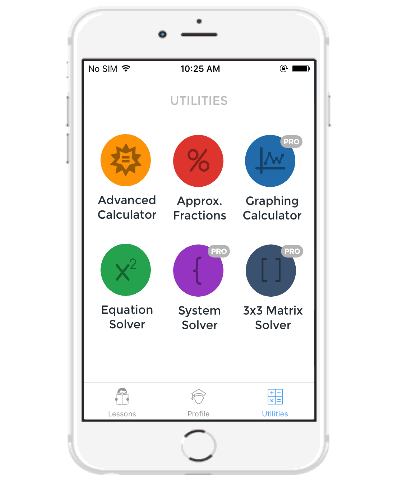Editor Ratings:
User Ratings:
[Total: 0 Average: 0]

iMathematics is a free Maths learning iPhone app that offers more than 70 topics scattered across 9 different lessons, like Arithmetic, Algebra, Plane Geometry, Trigonometry, Calculus, etc. Using this app, you’ll be able to easily learn mathematical lessons right on iPhone. This app offers dozens of topics related to equational problems, formulas, theorems, laws of trigonometry, the example of limits & continuity, probability concept, line segment, geometrical definitions & examples, and more at your fingertips. All the 70+ topics come with simple explanations which can help you quickly learn mathematical terms and how to exactly use them for solving maths problems. Some of the topics also offer quizzes which you can practice to improve your mathematical knowledge.

Apart from that, it also provides some utilities like an advanced calculator, Approx. Fractions finder, and equation (algebraic) solver. These utilities can assist you to solve mathematical problems while you’re going through the lessons. It also lets you track your progress in lessons as well as your weekly goals (sessions).Note: To use some additional feature like step-by-step solved exercise, 120+ topics, more interactive quizzes to practice, performance tracker, graphing calculator, system solver, 3×3 matrix solver, etc., you would need to upgrade to the PRO version of iMathematics.

## How to Learn Maths on iPhone using iMathematics:

To get started, simply open up the app and then the “Objectives” screen will appear where it will ask you to choose sessions per week for your goal either as casual (3 sessions), Regular (5 lessons), and Serious (7 sessions).After that, the main screen will appear where you’ll see all the mathematical lessons offered by this app on the “Lessons” tab. Currently it offers the following 9 lessons in the free version: Arithmetic, Algebra, Plane Geometry, Solid geometry, Analytic Geometry, Trigonometric Functions, Trigonometry, Calculus, and Probability.Each of the lesson offers various topics with explanations, examples, quizzes, etc., which can help you easily learn and solve mathematical problems. So, let us take a look at the topics offered by different lesson below.

• Arithmetic: It covers topics like Prime Numbers, Divisibility, Monomial & Polynomial, Sets, Complex Numbers, etc.
• Algebra: The “Algebra” lesson offers topics like Operation Properties, Powers, Factoring Polynomials, Linear Equations, Radicals, and more.
• Plane Geometry: This lesson covers topics such as Triangle, Right Triangles, Circle, Quadrilaterals, Polygons, and more.
• Solid Geometry: In this lesson, you can learn topics like Polyhedra, Cube, Cone, Sphere, Truncated Pyramid, etc.
• Analytic Geometry: The “Analytic Geometry” lesson comes with topics like Translation, Reflection, Rotation, Line Segment, Ellipse, and more.
• Trigonometric Functions: This lesson covers only the Trigonometric Functions – Right Triangle in the free version.
• Trigonometry: In the free version this lesson offers only two topics i.e. Law of Tangents and Solving Triangles.
• Calculus: You can learn topics like Limits, Continuity, Cauchy’s Mean Value Theorem, Integrals, Combinatorics, etc. in this lesson.
• Probability: This lesson covers basic concepts of probability, events, classical probability, addition rule, Bayes’ theorem, etc.

Apart from these useful lessons, this app also comes with some additional utilities like Equation Solver, Approx. Fractions Finder, and Advanced Calculator in the free version.Let me explain these utilities briefly for you.

• Advanced Calculator: This utility uses “Wolfram Alpha” computational knowledge engine to help you calculate mathematical problems.
• Approx. Fractions Finder: Using this utility tool, you can easily find the approx. fraction of any number with respect to the maximum possible denominator.
• Equation Solver: This utility lets you easily calculate quadratic equations in the form of ax2 + bx + c = 0.

## My Final Verdict:

iMathematics is a very useful app for iPhone which can assist you to learn maths with ease. The lessons  offered by this app are pretty useful and can definitely help you improve your mathematical knowledge. Going through the topics you can learn various mathematical terms and then easily solve problems of these terms. Go ahead and download the app right away to start learning maths on your iPhone.